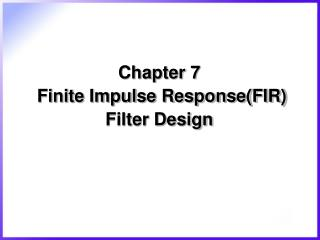# Chapter 7 Finite Impulse Response(FIR) Filter Design - PowerPoint PPT PresentationDownload PresentationChapter 7 Finite Impulse Response(FIR) Filter Design

Chapter 7 Finite Impulse Response(FIR) Filter DesignDownload Presentation## Chapter 7 Finite Impulse Response(FIR) Filter Design

- - - - - - - - - - - - - - - - - - - - - - - - - - - E N D - - - - - - - - - - - - - - - - - - - - - - - - - - -
##### Presentation Transcript

1. Chapter 7 Finite Impulse Response(FIR) Filter Design

2. 1. Features of FIR filter • Characteristic of FIR filter • FIR filter is always stable • FIR filter can have an exactly linear phase response • FIR filter are very simple to implement. Nonrecursive FIR filters suffer less from the effect of finite wordlength than IIR filters

3. 2. Linear phase response • Phase response of FIR filter • Phase delay and group delay (1) where (2)

4. Condition of linear phase response (3) (4) Where and is constant Constant group delay and phase delay response

5. If a filter satisfies the condition given in equation (3) • From equation (1) and (2) thus

6. It is represented in Fig 7.1 (a),(b)

7. When the condition given in equation (4) only • The filter will have a constant group delay only • It is represented in Fig 7.1 (c),(d)

8. Center of symmetry Fig. 7-1.

9. Table 7.1 A summary of the key point about the four types of linear phase FIR filters

10. Example 7-1 • Symmetric impulse response for linear phase response. No phase distortion

11. Frequency response where

12. (3) where

13. 3. Zero distribution of FIR filters • Transfer function for FIR filter

14. Four types of linear phase FIR filters • have zero at is real and is imaginary

15. If zero on unit circle • If zero not exist on the unit circle • If zeros on

16. Necessary zero Necessary zero Necessary zero Necessary zero Fig. 7-2.

17. 4. FIR filter specifications • Filter specifications peak passband deviation (or ripples) stopband deviation passband edge frequency stopband edge frequency sampling frequency

18. ILPF Satisfies spec’s Fig. 7-3.

19. Characterization of FIR filter • Most commonly methods for obtaining • Window, optimal and frequency sampling methods

20. 5. Window method • FIR filter • Frequency response of filter • Corresponding impulse response • Ideal lowpass response

21. Fig. 7-4.

22. Truncation to FIR • Rectangular Window

23. Fig. 7-5.

24. Fig. 7-6.

25. Fig. 7-7.

26. Table 7.2 summary of ideal impulse responses for standard frequency selective filters and are the normalized passband or stopband edge frequencies; N is the length of filter

27. Common window function types • Hamming window where N is filter length and is normalized transition width

28. Kaiser window where is the zero-order modified Bessel function of the first kind where typically

29. Kaiser Formulas – for LPF design

30. Example 7-2 • Obtain coefficients of FIR lowpass using hamming window • Lowpass filter Passband cutoff frequency Transition width Stopband attenuation Sampling frequency

31. Using Hamming window

32. Fig. 7-9.

33. Example 7-3 • Obtain coefficients using Kaiser or Blackman window Stopband attenuation passband attenuation Transition region Sampling frequency Passband cutoff frequency

34. Using Kaiser window

35. Fig. 7-10.

36. Summary of window method 1. Specify the ‘ideal’ or desired frequency response of filter, 2. Obtain the impulse response, , of the desired filter by evaluating the inverse Fourier transform 3. Select a window function that satisfies the passband or attenuation specifications and then determine the number of filter coefficients 4. Obtain values of for the chosen window function and the values of the actual FIR coefficients, , by multiplying by

37. Advantages and disadvantages • Simplicity • Lack of flexibility • The passband and stopband edge frequencies cannot be precisely specified • For a given window(except the Kaiser), the maximum ripple amplitude in filter response is fixed regardless of how large we make N

38. 6. The optimal method • Basic concepts • Equiripple passband and stopband • For linear phase lowpass filters • m+1 or m+2 extrema(minima and maxima) • Weighted • Approx. error Weighting function Ideal desired response Practical response where m=(N+1)/2 (for type1 filters) or m =N/2 (for type2 filters)

39. Practical response Ideal response Fig. 7-11.

40. Fig. 7-12.

41. Optimal method involves the following steps • Use the Remez exchange algorithm to find the optimum set of extremal frequencies • Determine the frequency response using the extremal frequencies • Obtain the impulse response coefficients